# 【附源码+Pybind + Cython Python性能提升方案】该拿什么拯救你，Slow Python

Cython介绍

Pybind11

Python

Cython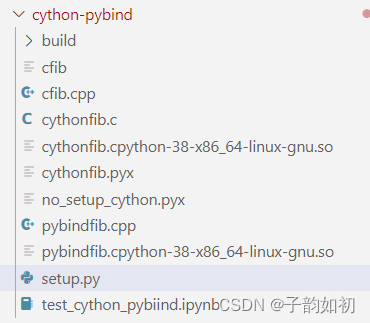# Cython介绍

1. 因为某些需求导致不得不编写一些多重嵌套的循环，而这些循环如果用 C 语言来实现会快几百倍，但是不熟悉 C 或者不知道 Python 如何与 C 进行交互

2. 项目组规定只能使用 Python 语言，解释器只能 CPython

3. Python 是一门动态语言，但希望至少在数字计算方面，能够加入可选的静态类型，这样可以极大的加速运算效果。因为单纯的数字相加不太需要所谓的动态性，尤其是当你的程序中出现了大量的计算逻辑时

4. 对于一些计算密集型的部分，写出一些超越 Numpy、Scipy、Pandas 的算法

5. 项目中有一些已经用 C、C++ 实现的库，需要直接在 Python 内部更好地调用它们，并且不使用 ctypes、cffi 等模块

6. 可以将 Python 代码中性能关键的部分使用 C 进行重写，来达到提升性能的效果。但是这需要对 Python 解释器有很深的了解，熟悉底层的 Python/C API，而这是一件非常痛苦的事情。

Cython将 Python 语言丰富的表达能力、动态机制和 C 语言的高性能汇聚在了一起，并且代码写起来仍然像写 Python 一样。

# 性能比对

## 纯C++

``````#include <time.h>

#include <iostream>
// g++ cfib.cpp -Ofast -o cfib && ./cfib
double cppfib(int n) {
int i;
double a = 0.0, b = 1.0, tmp;
for (i = 0; i < n; ++i) {
tmp = a;
a = a + b;
b = tmp;
}
return a;
}
int main() {
clock_t st, ed;
st = clock();
double r = cppfib(10000000);
ed = clock();
std::cout << (double)(ed - st) / CLOCKS_PER_SEC << std::endl;
std::cout << r << std::endl;
return 1;
}``````

``g++ cfib.cpp -o cfib && ./cfib``

22.23ms

``g++ cfib.cpp -Ofast -o cfib && ./cfib``

15.217ms

## Pybind11

``````#include <pybind11/pybind11.h>
// c++ -shared -rdynamic -fPIC -undefined  -fvisibility=hidden -std=c++14
// \$(python3 -m pybind11 --includes)  pybindfib.cpp -o pybindfib\$(python3-config
// --extension-suffix)
namespace py = pybind11;
double cppfib(int n) {
int i;
double a = 0.0, b = 1.0, tmp;
for (i = 0; i < n; ++i) {
tmp = a;
a = a + b;
b = tmp;
}
return a;
}
PYBIND11_MODULE(pybindfib, m) { m.def("cppfib", &cppfib); }``````

``c++ -shared -rdynamic -fPIC -undefined  -fvisibility=hidden -std=c++14``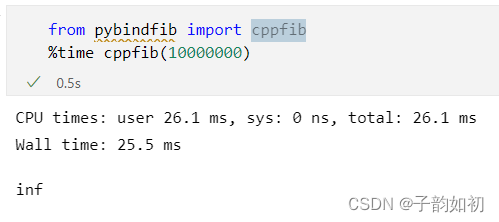## Python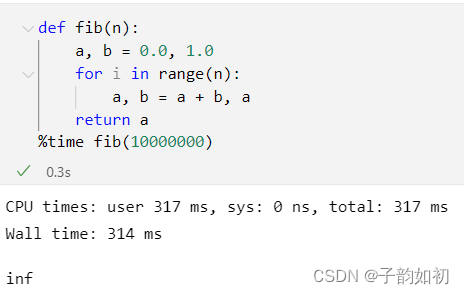## Cython

no_setup_cython.pyx

``````def fib(int n):
cdef int i
cdef double a = 0.0, b = 1.0
for i in range(n):
a, b = a + b, a
return a``````
``````cdef extern from "cfib.h":
double cfib(int n)``````

setup.py

``````from setuptools import setup
from Cython.Build import cythonize

setup(
ext_modules = cythonize("cythonfib.pyx")
)
# python setup.py build_ext --inplace``````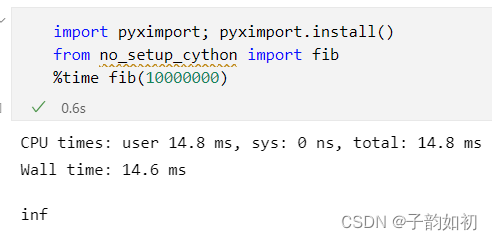# 总结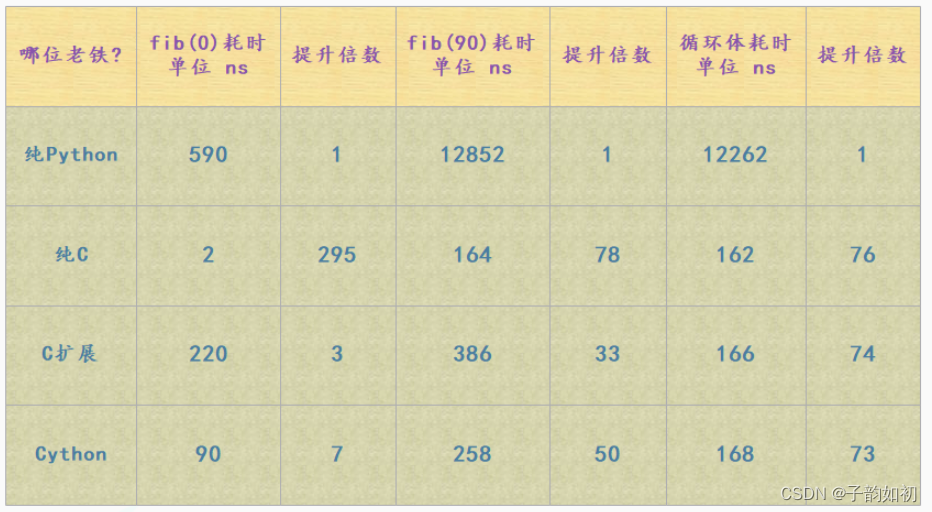## 循环机制

Python 在遍历一个可迭代对象的时候，会先调用这个可迭代对象内部的__iter__ 方法返回其对应的迭代器，然后再不断地调用这个迭代器的 __next__ 方法，将值一个一个的迭代出来，直到迭代器抛出 StopIteration 异常，for循环捕捉，终止循环。而迭代器是有状态的，Python 解释器需要时刻记录迭代器的迭代状态

## 算数操作

Python 由于其动态特性，使得其无法做任何基于类型的优化。比如：循环体中的 a + b，这个 a、b 指向的可以是整数、浮点数、字符串、元组、列表，甚至是我们实现了魔法方法 __add__ 的类的实例对象。尽管人为是知道是浮点数，但是 Python 不会做这种假设，所以每一次执行 a + b 的时候，都会检测其类型到底是什么。然后判断内部是否有 __add__ 方法，以及两者能不能相加，然后条件满足的话再调用对应的 __add__ 方法，将 a 和 b 作为参数，将 a 和 b 指向的对象进行相加。计算出结果之后，再返回其指针转成 PyObject * 返回

## 内存分配

Python 中的对象是分配在堆上面的，因为 Python 中的对象本质上就是 C 中的 malloc 函数为结构体在堆区申请的一块内存。在堆区进行内存的分配和释放是需要付出很大的代价的，而栈则要小很多，并且它是由操作系统维护的，会自动回收，效率极高。尽管 Python 引入了内存池机制使得其在一定程度上避免了和操作系统的频繁交互，并且还引入了小整数对象池以及针对字符串的intern机制。但事实上，当涉及到对象（任意对象、包括标量）的创建和销毁时，都会增加动态分配内存、以及 Python 内存子系统的开销。而 float 对象又是不可变的，因此每循环一次都会创建和销毁一次，所以效率依旧是不高的

## 更多情况

``````_temp/test.c:1047:18: warning: ‘__pyx_f_4test_gen’ defined but not used [-Wunused-function]
static PyObject *__pyx_f_4test_gen(int __pyx_v_x) {
t3.c:556:12: warning: ‘__pyx_f_2t3_fun’ defined but not used [-Wunused-function]
static int __pyx_f_2t3_fun(char *__pyx_v_s) {``````